# Curve toolbar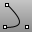Conic

Draw a conic section curve with options for the start, end, apex, and rho value.Conic, Perpendicular

Draw a conic curve perpendicular at the start.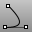Conic, Tangent at start

Draw a conic curve tangent at the start.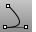Conic, Tangent at start and end

Draw a conic curve tangent at start and end.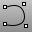Curve

Draw a curve from control point locations.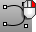CurveThroughPt

Fit a curve through point objects.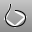CurveThroughPolyline, Control point

Creates a control-point curve though polyline vertices.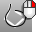CurveThroughPolyline, Interpolated

Creates an interpolated curve through polyline vertices.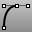HandleCurve

Draw chained Bézier curves with editing handles.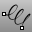Helix

Draw a helical curve with options for number of turns, pitch, vertical, reverse, and around a curve.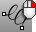Helix, Vertical

Draw a vertical helical curve.Hyperbola

Draw a hyperbolic curve from focus points, vertices, or coefficient.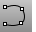InterpCrv

Fit a curve through picked locations.HandleCurve

Draw chained Bézier curves with editing handles.InterpCrvOnSrf

Fit a curve through locations on a surface.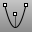Parabola, Focus

Draw a parabolic curve from focus points.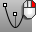Parabola, Vertex

Draw a parabolic curve from vertex and end.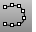Sketch

Drag the mouse to draw a curve.Sketch, on surface

Drag the mouse to draw a curve on a selected surface.Sketch, on mesh

Drag the mouse to draw a curve on a selected mesh object.Spiral

Draw a spiral curve with options for number of turns, pitch, flat, vertical, and around a curve.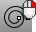Spiral, Flat

Draw a flat spiral curve.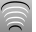TweenCurves

Create curves between two open or closed input curves.

Rhinoceros 5 © 2010-2015 Robert McNeel & Associates. 17-Sep-2015